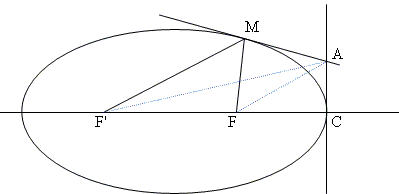## Urquhart's Theorem For And By Conics

Urquhart's theorem states that, for straight lines ABB' and AC'C with BC and B'C' intersecting in D the relation AB + BD = AC' + C'D implies AB' + B'D = AC + CD, as when four lines displayed all touch the same circle.Michel Cabart wondered whether an "hyperbolic" version of the theorem is also true:

 Is BD - BA = C'D - C'A equivalent to B'D - B'A = CD - CA?

What follows is the combination of Michel's emails of December 9 and 15, 2008.

Using a trigonometric approach in analogy to the "circular theorem":

 (BD - BA) / AD = (sin2β' - sin2β) / sin2(β + β') = [sin(β' - β) cos(β' + β] / sin(β' + β) cos(β' + β) = sin(β' - β) / sin(β' + β) = [sinβ' cosβ - sinβ cosβ'] / [sinβ' cosβ + sinβ cosβ'] = (t - 1) / (t + 1)

by posing t = tanβ' / tanβ. Thus BD - BA = C'D - C'A iff tanβ' / tanβ = tanγ / tanγ' and the rest of the proof is similar.

The two variants of the theorem can be summarized into one single property: "If B and C' belong to an ellipse (resp. hyperbola), then B' and C also belong an ellipse (resp. hyperbola) with the same foci."

This suggests a proof by conics theorems. The following one (detailed for ellipse and similar for hyperbola) uses the following property of the focuses: for any two tangents MA and CA intersecting in A, FA bisects MFC and F'A bisects MF'C. Here we take CA perpendicular to the major axis.Notations:

• ∠ MF'C = 2α' and ∠MFC = 2α,
• c = half the distance between foci, a = half the length of the major axis.

We then have:

 tanα' = AC / (a + c) and tanα = AC / (a - c)

so that

 tanα' / tanα = (a - c) / (a + c)

Thus returning to the problem: BD + BA = C'D + C'A iff

 tanβ' / tan(π/2 - β) = (a - c) / (a + c) = tanγ / tan(π/2 - γ').

which is the condition found by trigonometric calculations.### Urquhart's Theorem